# Anyone Can Learn Anything

## Let’s draw a cool plot 1

In this post, we will learn how to draw a cool plot. Although there are many good graph packages in R, it is good to know how to plot using base commands. The very first thing that we need to know is not a histogram, or a bar-chart, but just a x-y plot. This is the perfect example when you study commands used for plotting in R.

Let us assume that we have two vectors: one is x vector and the other one is y vector which is generated by using x vector as follows. We want to draw a plot of $y=x^2$.

x <- seq(-10, 10, length.out = 100)
y <- x^2

To draw a plot of x and y, let us type the following code in R:

plot(x,y)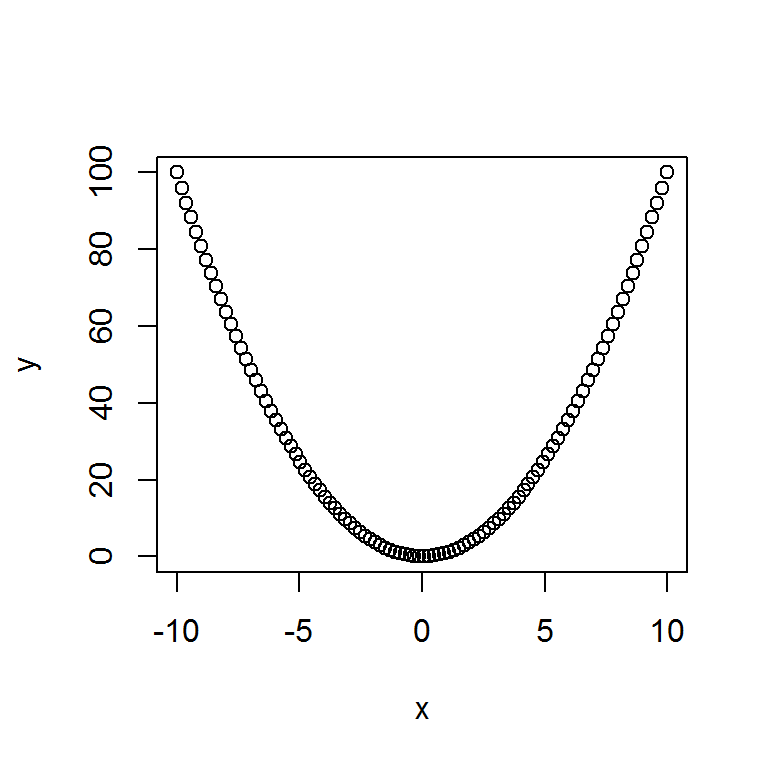Let us make it look cooler.

First, I want to change the points into a line since I intend to get a plot of $y=x^2$. Also, the color of the line should be different than black because of the coolness.

plot(x,y, type = 'l', col = "Blue")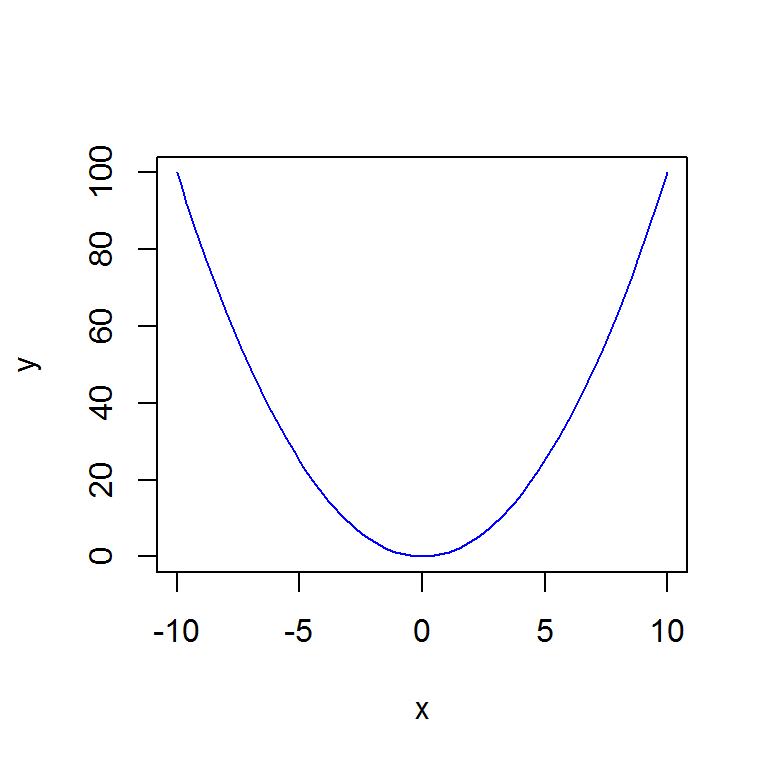Second, there is no need to show the whole part of the graph since I want to check the y values around zero. Thus, the range of the plot should be adjusted. Here, I set the range of the x axis from -2 to 2 and the range of the y axis from 0 to 4, which is set corresponding to the x values; the maximum value of the squared x values is 4 and the minimum is 0.

plot(x,y, xlim = c(-2,2), ylim = c(0,4), type = 'l', col = "Blue")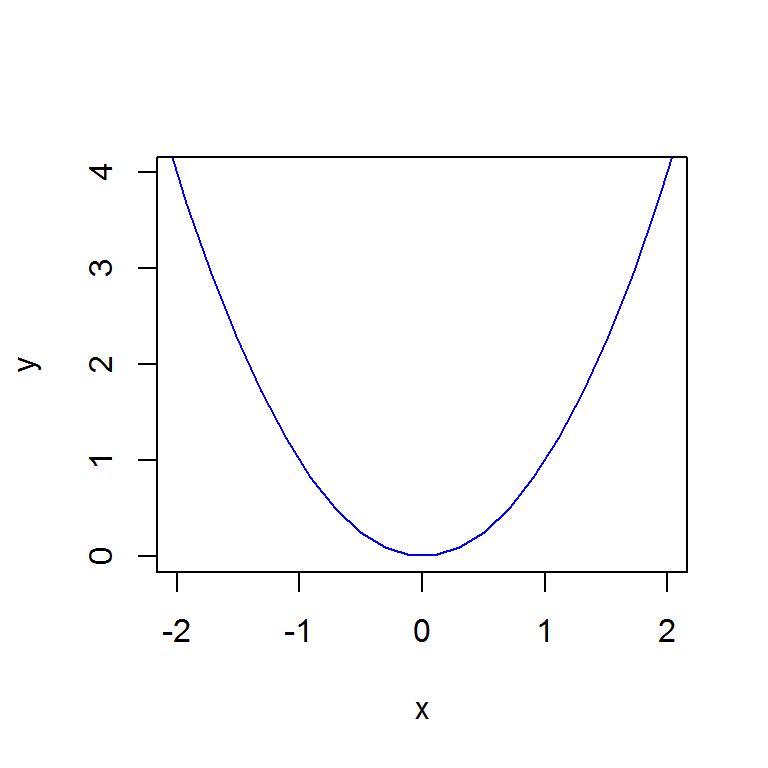Third, we didn’t draw the x and y axes, that is not cool.

plot(x,y, xlim = c(-2,2), ylim = c(0,4), type = 'l', col = "Blue")
abline( v = 0, lty = 1)
abline( h = 0, lty = 1)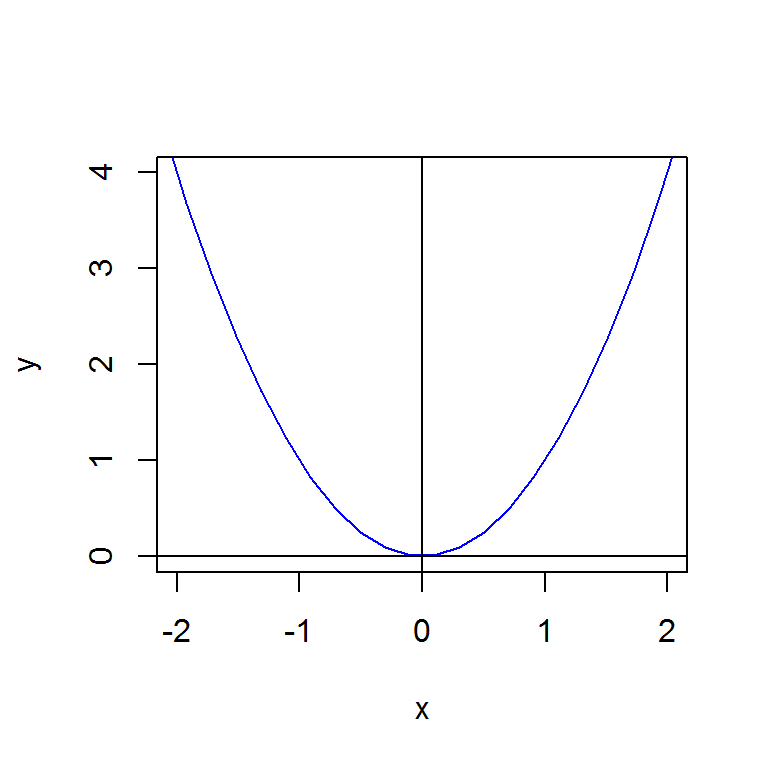The abline command allows us to add straight lines to the generated plot object. The syntax of abline is as follows:

abline(a = NULL, b = NULL, h = NULL, v = NULL, reg = NULL,
coef = NULL, untf = FALSE, ...)

Arguments a and b indicate the intercept and slope. Arguments h and v indicate the y-value for the horizontal line and the x-value for the vertical line respectively. Thus, the first used command, abline( v = 0, lty = 1), is for the y axis and the second used command, abline( h = 0, lty = 1), is for the x axis. Argument ‘lty’, which stands for line type, is used for choosing the type of lines; when we set the argument as 1 or 2, the solid line or dotted line will appear in the plot respectively. Once we understand abline, we can make our plot more accurate as follows:

plot(x,y, xlim = c(-2,2), ylim = c(0,4), type = 'l', col = "Blue")
abline(h = 0:4, v = -2:2, col = "Gray", lty=3)
abline( v = 0, lty = 1)
abline( h = 0, lty = 1)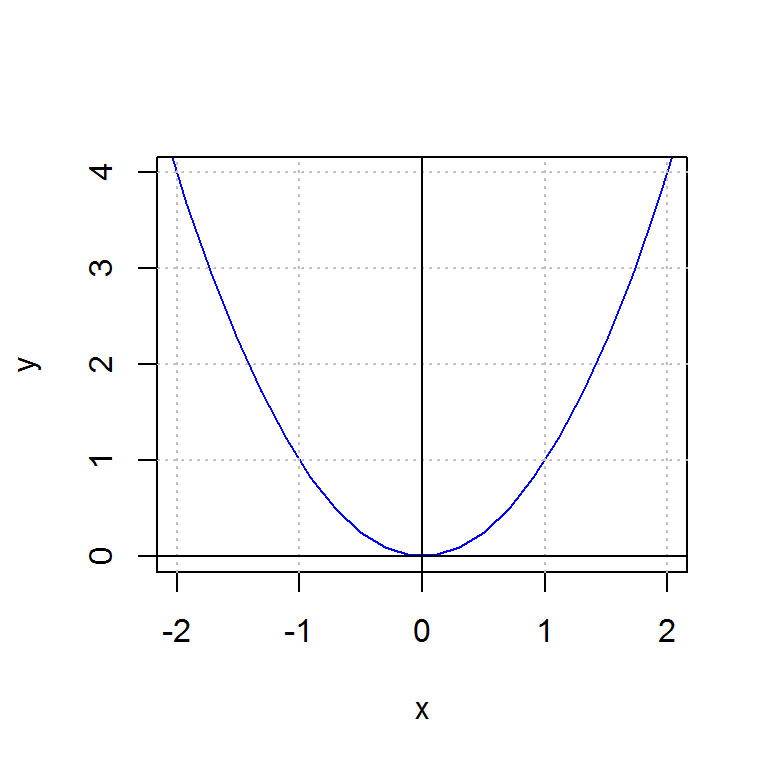Note that we draw the gray lines first and then draw the x and y axes. Otherwise, we can’t see the axes since they would overlaid by the gray lines. The reader also can see that ten gray dotted lines are added by just using one line command, abline(h = 0:4, v = -2:2, col = “Gray”, lty=3), which comes from the vectorized property of R.

#### 'R Programming > Graphics' 카테고리의 다른 글

 ggplot2 패키지 정리 - 1. stat_density, stat_function, geom_rug, geom_point, anotate, labs  (0) 2017.07.11 2017.05.28 2017.05.28 2017.05.28 2017.05.28 2017.05.28 2017.05.24 2017.05.24 2017.01.11 2017.01.10